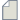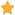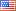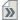###Author Topic: Total energy at a different broadening?  (Read 126 times)

0 Members and 1 Guest are viewing this topic.

####k.cerda

• Regular ATK user
•• Posts: 10
• Country:• Reputation: 0##### Total energy at a different broadening?
« on: September 26, 2021, 05:47 »
Hello

The total energy gives the total energy  ('Total free energy' at T<> 0 K) and the ground state energy (i.e., at T=0 K). The difference is σS. Is it possible to calculate the total free energy at a broadening other than T=0, e.g. at σ1 using a correction term such as σ1S? Thanks.

####filipr

• Regular ATK user
•• Posts: 14
• Country:• Reputation: 3
• QuantumATK developer##### Re: Total energy at a different broadening?
« Reply #1 on: September 27, 2021, 17:47 »
Actually, the TotalEnergy analysis object in QuantumATK by default gives the total free energy at the electronic temperature/broadening specified when doing the ground state calculation. The extrapolated total energy at T = 0 K has to be obtained either from the text output in the log or from using:
Code
``````energy_at_zero_kelvin = total_energy.alternativeEnergies()['Zero-Broadening-Energy']
``````
If you want the free energy at a different energy you have to repeat the calculation, changing the broadening under the "Numerical Accuracy" settings. If you for some reason want to extrapolate to a broadening different than the one you did the calculation for you can simply use that F(σ) = E(0) + 1/2 γσ2 + O(σ4), i.e. it is approximately a parabola. You have two points on this parabola: E(0) and F(σ) at the σ used in the calculation - from those you can extrapolate to any other σ in a close neighborhood. See also: https://docs.quantumatk.com/manual/Types/TotalEnergy/TotalEnergy.html and https://docs.quantumatk.com/manual/technicalnotes/occupation_methods/occupation_methods.html

####k.cerda

• Regular ATK user
•• Posts: 10
• Country:• Reputation: 0##### Re: Total energy at a different broadening?
« Reply #2 on: September 28, 2021, 13:46 »